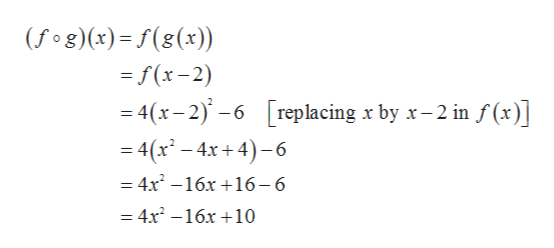# 20. Let f(x) = 4x2 – 6 and g(x) = x– 2.(a) Find the composite function ( f og)(x) and simplify. Show work.(b) Find ( f og)(−1) . Show work.

Question
42 views

20. Let f(x) = 4x2 – 6 and g(x) = x– 2.
(a) Find the composite function ( f og)(x) and simplify. Show work.
(b) Find ( f og)(−1) . Show work.

check_circle

Step 1

Given,

Step 2

a). The composite f...help_outlineImage Transcriptionclose(fog)(x)= f(8(x)) =f(x-2) =4(x-2)-6 replacing x by x-2 in f(x)] -4(r-4x+4)-6 = 4x-16x 16-6 = 4x-16x10 fullscreen

### Want to see the full answer?

See Solution

#### Want to see this answer and more?

Solutions are written by subject experts who are available 24/7. Questions are typically answered within 1 hour.*

See Solution
*Response times may vary by subject and question.
Tagged in

### Functions